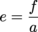Study Guide

# Conic Sections - General Conic Equation and Eccentricity

## General Conic Equation and Eccentricity

If we put a Chihuahua and a Great Dane side by side, they obviously look a little different. At the same time, we have no problem calling them both dogs. It's the same thing with the conic sections. They all look different, but we still recognize them as the same type of thing.

All of the different conic sections actually have even more in common than we've seen so far. We've treated them as though they all have different equations, but in fact there is only 1 equation for all the conics. If you don't believe us, check it out: the general conic equation.

Ax2 + Bxy + Cy2 + Dx + Ey + F = 0

Here A, B, C, D, E and F are the constants. They don't look too much like any of the equations we've worked with. But looks can be deceiving.

The first thing we'll say is that B has always been 0 for every conic we've looked at so far, and it's going to stay that way. x and y multiplied together is a recipe for headaches. If you've wondered why all of our conics have mysteriously been parallel to the x- or y-axes in some way, B = 0 is why. B is what rotates a conic around, so we haven't messed with that at all.

Let's look at the other variables, especially A and C. Suppose one of them, let's go with A, equaled 0. Then we'd have:

Cy2 + Dx + Ey + F = 0

With a little rearranging, we could write this as:

Dx + F = -Cy2 + Ey

That's just a little bit of completing the square away from being a parabola; a single x term equal to some y2 term. We could do this kind of thing and find all of the conic sections, but we're not gonna. We'll just go ahead and summarize the results for you. B is assumed to be 0 for all of these.

• If either A = 0 or C = 0, but not both of them, then we have a parabola.
• If A = C, and they both aren't 0, then we have a circle.
• If AC, and AC > 0, then we have an ellipse.
• If AC, and AC < 0, then we have a hyperbola.

You see, we really only need to concern ourselves with A and C, the constants attached to x2 and y2. But what's with checking if AC is greater than or less than 0? It's the sign, silly. If A and C are both positive or both negative, then AC is positive and the conic will be an ellipse. If one of them is negative, then AC is negative and we have a hyperbola.

So, all of the conics have more in common than just a name. Good to know. But now we have a surprise; we have another way to define the conics and tell them apart. Doesn't that make just want to get up and dance? Probably not, but do you really need an excuse to dance? Probably. We'll just move on, then.

### They're Not Odd, They're Just Eccentric

Just as it seems like we've defined every possible way of identifying a conic section, we’re throwing in another. The eccentricity is a ratio of two different parts of a conic.f is the distance from the center to the focus / foci. We've seen this guy a lot before. We did call it p back when we were working with parabolas, though. a is the distance from the center to vertex / vertices, or the length of the semi-major axis in ellipses. The names, or the exact meaning of them, has changed from one type of conic to the next, but we're familiar with all of them by now.

That's a good thing, too, because we can use the eccentricity to define the conics. Check it:

• If e = 0, we have a circle. The focus and the center are right on top of each other, for a distance of 0.
• If e is greater than 0, but less than 1, we have an ellipse. We've already mentioned that the foci are inside of an ellipse, so f < a.
• If e = 1, then we have a parabola. Parabolas don't have a center, but we know that p is the distance from the vertex to both the focus and the directrix, so we can kinda see where this result comes from.
• If e is greater than 1, then we have a hyperbola. The focus is farther from the center than the vertex, so that works out. However, notice that the a in the eccentricity formula may not be a from the hyperbola formula. We want the distance to the vertex, which is given by b in a vertical hyperbola. Proceed with caution.

Each conic section is determined by how eccentric it is. The circle is the least eccentric, a very well rounded individual, while the hyperbola is the most eccentric, like the uncle who collects different styles of Christmas wrapping paper.

Oh hey, we think we're done. We don't have anything else to say about conic sections. Good night everybody.